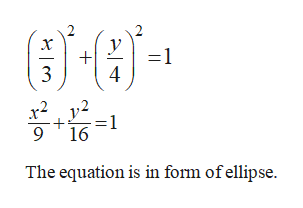# Describe the motion of a particle with position (x, y) as t varies in the given interval.x = 3sin(t)y = 4cos(t)-π ≤ t ≤ 6π

Question
3 views

Describe the motion of a particle with position (x, y) as t varies in the given interval.
x = 3sin(t)
y = 4cos(t)
-π ≤ t ≤ 6π

check_circle

Step 1

To find: The motion of particle.

The expression is rewritten as,

Step 2

Substitute the values in formula, sin2(x)+cos2(y) = 1,help_outlineImage Transcriptionclosex y 1 4 3 y2 -1 16 9 The equation is in form of ellipse fullscreen
Step 3

Substitute the initial point of t interval in x(t)...

### Want to see the full answer?

See Solution

#### Want to see this answer and more?

Solutions are written by subject experts who are available 24/7. Questions are typically answered within 1 hour.*

See Solution
*Response times may vary by subject and question.
Tagged in

### Other